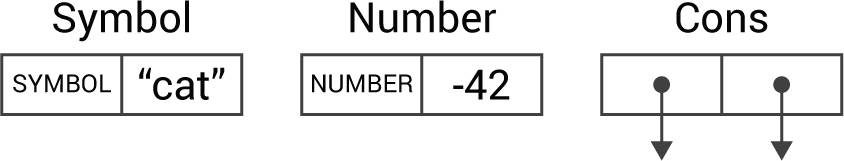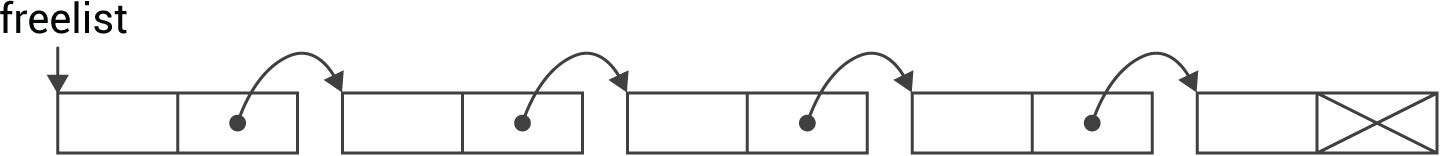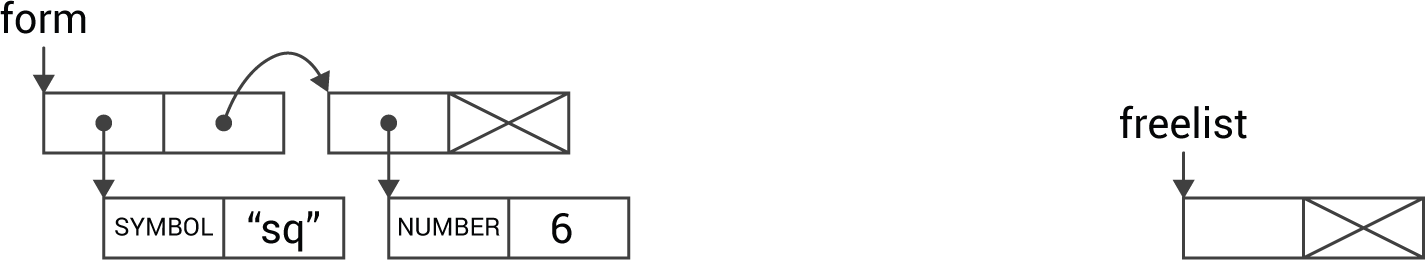## Objects

The following description refers to the 16-bit versions of uLisp. The 32-bit versions are essentially the same, except that objects consist of two 32-bit cells.

All objects in uLisp consist of two 2-byte cells, so every object occupies 4 bytes. There are three fundamental types of object: symbol, number, or cons:• A symbol contains the identifier SYMBOL in the left cell, and the symbol in the right cell.
• A number contains the identifier NUMBER in the left cell, and the 16-bit signed integer value in the right cell.
• Finally, a cons contains an address pointer in each cell pointing to other objects.

The type identifiers SYMBOL and NUMBER are defined by the type enum; a simplified version is:

`enum type {ZERO=0, SYMBOL=2, NUMBER=6};`

The low number addresses are never used, so we can uniquely identify the type from the left-hand cell.

The object type is defined by the following typedef:

```typedef struct sobject {
union {
struct {
sobject *car;
sobject *cdr;
};
struct {
unsigned int type;
union {
symbol_t name;
int integer;
int chars; // For strings
};
};
};
} object;```

The two pointers in a cons cell are called car and cdr, for historical reasons.

### Setting up uLisp's workspace

The space used by uLisp is reserved by the declaration:

`object Workspace[WORKSPACESIZE] OBJECTALIGNED;`

The workspacesize depends on the amount of RAM available on the processor. It's 318 objects on an ATmega328.

Initially all the workspace is allocated to the freelist: a list of objects, linked together by cdr pointers. The last object in the list has a NULL cdr pointer:The workspace is initialised by initworkspace():

```void initworkspace () {
Freelist = NULL;
for (int i=WORKSPACESIZE-1; i>=0; i--) {
object *obj = &Workspace[i];
car(obj) = NULL;
cdr(obj) = Freelist;
Freelist = obj;
Freespace++;
}
}```

### Making objects

When allocating objects, cells are taken from the front of the freelist, and the freelist pointer is updated. For example, after creating the list:

`(sq 6)`

the freelist will be:An object is allocated by calling myalloc():

```object *myalloc () {
if (Freespace == 0) error2(NIL, PSTR("no room"));
object *temp = Freelist;
Freelist = cdr(Freelist);
Freespace--;
return temp;
}```

The myalloc() function is called by the routines that make an object of each type.

Number objects are created by number(), which takes an integer value:

```object *number (int n) {
object *ptr = myalloc();
ptr->type = NUMBER;
ptr->integer = n;
return ptr;
}```

Symbol objects are created by symbol(), which takes an unsigned integer containing a packed version of the symbol:

```object *symbol (symbol_t name) {
object *ptr = myalloc();
ptr->type = SYMBOL;
ptr->name = name;
return ptr;
}```

Finally cons() takes two objects to be pointed to by the car and cdr of the cons:

```object *cons (object *arg1, object *arg2) {
object *ptr = myalloc();
ptr->car = arg1;
ptr->cdr = arg2;
return ptr;
}```

Previous: Implementation

Next: Symbols